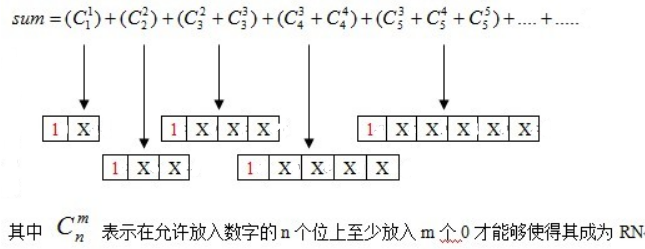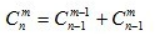# POJ 3252 - Round Numbers

## 问题描述

• 转换所得的二进制数，最高位必然是1，最高位的前面不允许有0
-规定输入范围： `1 <= a < b <= 2E`

## 解题思路

• （1）规定输入范围：`1 <= a < b <= 2E`

输入的数是一个接近大数的非大数, int可以存储。网上看很多同学都说要用到精度，其实完全没必要，int能表示21E+的整数，精确的int极限能表示的正整数为2147483647，但是即使这样，面对这题也不能松懈啊！ `bin[]` 边界的最小值为 35 ！！
• （2）`bin[]` 数组若果定义为局部数组，等着WA吧！

我找不到任何原因为什么会这样，bin不管是全局定义 还是 局部定义，本地是完全AC的，上传就出问题了，局部WA，全局AC。

我被迫把传参del掉，把bin改为全局，郁闷！
• （3）组合数打表，同(1)的猥琐，`c[][]` 边界的最小值为33，就是说如果定义组合表的大小比 `c` 小的，就等着RE吧!

还有就是这个算法有一个违背常识的处理，要把 `c=1`，不然某些最终结果会少1
• （4）输入不能用循环输入 `while(cin>>…)` ，不然你就等着OLE (就是Output Limit Excessed，很少见吧！)。不知道数据库是怎么回事，输入竟然不会根据读取数据结束而结束，而是无限输出最后一次输入所得的结果……老老实实一次输出就end file吧！

• 闭区间 `[0, a]` 内有T个RN
• 闭区间 `[0, b+1]` 内有S个RN
• 再用 `S – T` 就是闭区间 `[a, b]` 内的RN数了

``````for(i=1;i<bin-1;i++)         //bin记录的是二进制数的长度len
for(j=i/2+1;j<=i;j++)
sum+=c[i][j];``````1表示当前处理的二进制数的最高位，X表示该二进制数待放入数字的位。

``````int zero=0;  //从高位向低位搜索过程中出现0的位的个数
for(i=bin-1;i>=1;i--)
if(bin[i])   //当前位为1
for(j=(bin+1)/2-(zero+1);j<=i-1;j++)
sum+=c[i-1][j];
else
zero++;``````

• （1）当前位置i后面允许组合的低位有多少个，我的程序由于bin是从 `bin` 开始存储二进制数的，因此 当前位置i后面允许组合的低位有 `i-1`

• （2） 组合前必须要除去前面已出现的0的个数zero## AC 源码

``````//Memory Time
//224K   16MS

#include<iostream>
using namespace std;

int c={0};
int bin;  //十进制n的二进制数

/*打表，计算nCm*/

void play_table(void)
{
for(int i=0;i<=32;i++)
for(int j=0;j<=i;j++)
if(!j || i==j)
c[i][j]=1;
else
c[i][j]=c[i-1][j-1]+c[i-1][j];
//    c=0;
return;
}

/*十进制n转换二进制，逆序存放到bin[]*/

void dec_to_bin(int n)
{
bin=0;   //b是二进制数的长度
while(n)
{
bin[++bin]=n%2;
n/=2;
}
return;
}

/*计算比十进制数n小的所有RN数*/

int round(int n)
{
int i,j;
int sum=0;  //比十进制数n小的所有RN数
dec_to_bin(n);

/*计算长度小于bin的所有二进制数中RN的个数*/

for(i=1;i<bin-1;i++)
for(j=i/2+1;j<=i;j++)
sum+=c[i][j];

/*计算长度等于bin的所有二进制数中RN的个数*/

int zero=0;  //从高位向低位搜索过程中出现0的位的个数
for(i=bin-1;i>=1;i--)
if(bin[i])   //当前位为1
for(j=(bin+1)/2-(zero+1);j<=i-1;j++)
sum+=c[i-1][j];
else
zero++;

return sum;
}

int main(void)
{
play_table();

int a,b;
cin>>a>>b;
cout<<round(b+1)-round(a)<<endl;

return 0;
}``````

目录